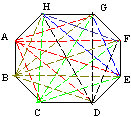Email us to get an instant 20% discount on highly effective K-12 Math & English kwizNET Programs!

#### Online Quiz (WorksheetABCD)

Questions Per Quiz = 2 4 6 8 10

### MEAP Preparation - Grade 6 Mathematics4.23 Diagonals of Polygons

 The number of diagonals in a polygon = 1/2 N(N-3), where N is the number of sides of a polygon Example: How many diagonals does a hexagon have?The number of diagonals in a polygon = 1/2 N(N-3) Here N = 6 = 1/2 x 6 (6-3) = 3 x 3 = 9 Answer: 9 Directions: Answer the following questions. Also draw draw and calculate how many diagonals does triangle, rectangle, square, parallelogram, trapezoid, rhombus, kite, pentagon, hexagon, heptagon, octagon, nonagon and decagon have.
 Q 1: How many diagonals does a hexagon have?9810 Q 2: How many diagonals does a rectangle have?243 Q 3: How many diagonals does a parallelogram have?324 Q 4: How many diagonals does a rhombus have?423 Q 5: How many diagonals does a octagon have?202119 Q 6: How many diagonals does a heptagon have?141516 Question 7: This question is available to subscribers only! Question 8: This question is available to subscribers only!